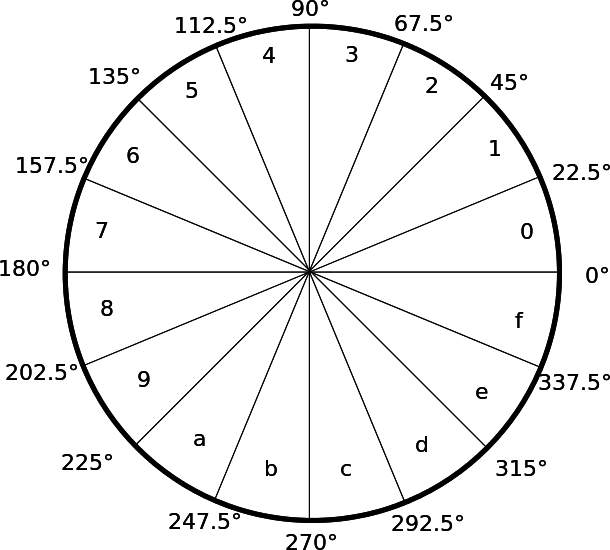시간 제한 메모리 제한 제출 정답 맞은 사람 정답 비율
1 초 1024 MB 10 4 2 25.000%

## 문제

In the film The Martian, astronaut Mark Watney is stuck on Mars, and communicates with earth by rotating a camera on an old space prod.

The prod points at a hexidecimal digit, i.e. 0-9 or a-f, and Mark creates letters and other characters by converting a pair of hexadecimal digits according to ASCII encoding. To determine which digit the prod is pointing at Mark has divided a circle of 360 degrees into 16 parts of 22.5 degrees each.For example, the pair of angles $(100*{\circ}, 30^{\circ})$ represents the hexadecimal number 41, which in decimal is $65$. According to ASCII that is the character A.

Mark is getting tired of writing down the digits manually, and he asks you to help him decode the messages he receives. You will be given the sequence of angles the prod points at and you're expected to print the message.

## 입력

The first line consists of an integer $2 \le N \le 50\,000$, the number of angles. $N$ will be even.

The following $N$ lines contain angles, one angle per line. An angle is given in degrees as a float in the range $[0, 360)$. The prod will never point at an angle which is exactly inbetween two hexadecial digits.

When decoding to ASCII only characters with decimal values 32-126 occurs.

## 출력

Print one line with the decoded message.

## 예제 입력 1

54
91
118
157
23
167
66
158
100
157
189
47
21
172
98
139
353
59
10
139
314
146
23
160
48
162
70
63
316
55
21
94
95
144
353
53
11
166
203
147
339
158
115
46
3
157
83
149
339
160
18
177
218
69
339


## 예제 출력 1

Earth to mars. Do you copy?


## 출처• 문제를 만든 사람: Johan Sannemo